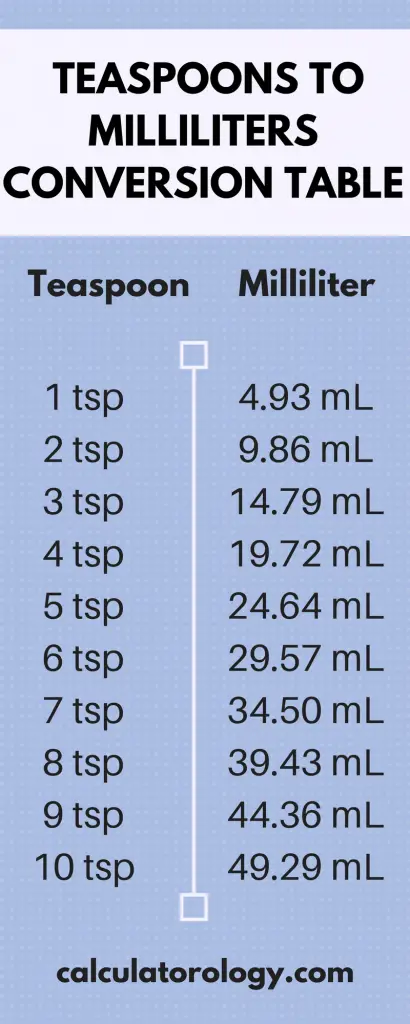код Teaspoon to Milliliter - Calculatorology

## Teaspoons to Milliliters Conversion

Converter

Enter a value that you want to convert into milliliters and click on the "convert" button.

### Teaspoon to Milliliters (How many milliliters in a teaspoon?)

A teaspoon is a volume unit that is often used in prescriptions and cooking recipes. It is abbreviated as "tsp" or "ts". We use small letters when abbreviating the teaspoon to avoid it being mistaken for a larger unit i.e. Tablespoon. One US teaspoon is equivalent to 1/6 of fluid ounces and 5 milliliters. A milliliter is a volume unit in the metric system abbreviated as "mL" or "ml". The conversion result depends on the standard used in the conversion.

##### Conversion

We multiply the value in teaspoon by the conversion factor to determine the number of milliliters in a teaspoon. According to the conversion factors;

1 Teaspoon [US] = 4.92892159 Milliliters

1 Teaspoon [Metric, Australia] = 5 milliliters

1 Teaspoon [UK] = 5.91938802 Milliliters

The unit equivalent of teaspoon and milliliter can also be multiplied with the base unit factor of volume cubic meter. The base unit factor of volume cubic meter for the teaspoon is 4.928922 × 10 -6 m³, while the milliliter [US] is 1 x 10-6 m3. Therefore, the calculation in the US standard will be expressed as;

1 Teaspoon = 4.928922 * 10-6 m³

1 mL = 1 * 10-6 m³

1 mL = 1.0E-6 m³

1 m³ = (1/1.0E-6) mL

1 m³ = 1000000 mL

1 Teaspoon = 4.928922 * 10-6 * 1000000 mL

1 Teaspoon = 4.928922 mL#### How many milliliters are in a teaspoon?

Before we determine the number of milliliters present in a teaspoon, it is essential to specify the standard for conversion. For the US teaspoon, one teaspoon is equal to 4.92892159 milliliters. The Metric and Australian teaspoons are equivalent to 5 milliliters. If you are using the UK standard, One teaspoon [UK] is equal to 5.91938802 Milliliters. We use the same method of calculation for other standards if you want to determine the number of milliliters in a single teaspoon. However, their conversion factors will differ depending on the standard you are using in the calculation.

The most common values are outlined in the volume conversion tables for quick reference. The Teaspoon to Milliliter conversion calculator is useful when performing long calculations or using larger values. Use the blank text field to enter the value teaspoons and then click the ‘Convert' button. The conversion result in milliliters is displayed immediately at the bottom platform of the calculator. Although it can only execute a single conversion at a time, you can always readjust the converter using the ‘Reset' button.